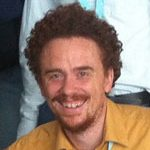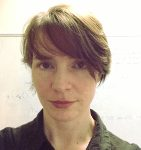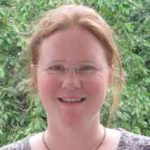# SpeakersJeremy Blanc (Universität Basel, Switzerland) Title: Automorphisms of P1 bundles over rational surfaces Abstract: In this talk, I will present a way to compute automorphisms of P1-bundles over rational surfaces. As it will be explained, we can reduce to the case of Hirzebruch surfaces, and then study the action on the fibres in an explicit way, using transition functions. Joint work with Andrea Fanelli and Ronan Terpereau.Jaroslaw Buczynski (University of Warsaw, Poland) Title: Constructions of k-regular maps using finite local schemes Abstract: A continuous map Rm -> RN or Cm -> CN is called k-regular  if the images of any k distinct points are linearly independent. Given integers m and k a problem going back to Chebyshev and Borsuk is to determine the minimal value of N for which such maps exist.  The methods of algebraic topology provide lower bounds for N, however there are very few results on the existence of such maps for particular values m. During the talk, using the methods of algebraic geometry, we will construct k-regular maps. We will relate the upper bounds on the minimal value of N with the dimension of the a Hilbert scheme. The computation of the dimension of this space is explicit for k< 10, and we provide explicit examples for k at most 5. We will also provide upper bounds for arbitrary m and k. The problem has its interpretation in terms of interpolation theory: for a topological space X and a vector space V, a map X -> V is k-regular if and only if the dual space V* embedded in the space of continuous maps from X to the base field R or C is k-interpolating, i.e. for any k distinct points x1,…,xk of X and any values fi, there is a function in V*, which takes values fi at xi.  Similarly, we can interpolate vector valued continuous functions, and analogous methods provide interesting results. The talk is based mainly on: – “Constructions of k-regular maps using finite local schemes”, Jarosław Buczyński, Tadeusz Januszkiewicz, Joachim Jelisiejew, Mateusz Michałek, to appear in Journal of European Mathematical Society, arXiv:1511.05707 – “Examples of k-regular maps and interpolation spaces, Mateusz Michałek, Christopher Miller, arXiv:1512.00609 – Follow up work in progress with Tadeusz Januszkiewicz.Eimear Byrne (University College Dublin, Ireland) Title: Fundamental Operations on Rank Metric Codes Abstract: We outline recent progress in rank-metric coding theory, making reference to the classical theory of Hamming metric codes. In the history of Hamming metric codes, fundamental coding theoretic operations such as puncturing and shortening have played an important role in questions of code optimality and code constructions. They have also arisen in the literature on the zeta function of a Hamming metric code (Duursma 2001). Rank-metric codes have featured prominently in the literature on algebraic codes in recent years. They have applications to error-correction in multicast networks and have been considered in code-based cryptosystems. Such codes are subsets of the matrix ring Fq(m x n) endowed with the rank distance function, which measures the Fq-rank of the difference of a pair of matrices. A small number of families of optimal rank metric codes have been known for some decades (Delsarte 1978, Gabidulin 1985, Roth 1991). These are examples of maximum rank distance (MRD) codes. Very recently, more general families of MRD codes have been discovered (Sheekey 2016) and there has been much activity in research into such codes. However, the general theory of rank metric codes is still very much open. We describe properties of shortened and punctured codes for matrix codes, with respect to the rank metric. We introduce a number of ways to define such operations and give a duality result. We use the notion of a rank metric shortened code to describe the rank-metric zeta function of a code in terms of a generating function and relate this object to the rank-metric weight enumerator of a code. We show that, as in the Hamming metric case, the normalized weight enumerator of a rank-metric code is invariant under shortening and puncturing. We outline the differences between the Hamming and rank metric theories on this topic. We discuss the rank-metric covering problem and introduce bounds on the rank metric covering radius. We show how these results can be applied to the classification of MRD and quasi-MRD codes. We describe several open problems in the theory of rank-metric codes.Lothar Göttsche (International Centre for Theoretical Physics Trieste, Italy) Title: Virtual refinements of the Vafa-Witten formula Abstract: Based of physics arguments Vafa and Witten made predictions about the Euler numbers of moduli spaces of sheaves on surfaces. They give explicit generating functions in terms of modular forms. These moduli spaces are in general very singular, but they have a perfect obstruction theory, and thus a virtual fundamental class and a virtual Tangent bundle, and thus virtual Chern numbers and in particular a virtual Euler number. In a virtual sense they are smooth projective varieties and carry the virtual versions of the invariants of smooth projective varieties. We interpret the Vafa-Witten prediction as being about the virtual Euler numbers. Then a formula of Mochizuki allows to compute the virtual Euler numbers in terms of integrals on Hilbert schemes of points, which we carry out via reduction to toric surfaces and applying Atiyah-Bott localization. These computations are carried out with a suitable program. This allows to check the conjecture in a wide variety of cases up to high expected dimensions of the moduli spaces. Further computations allow us to extend the conjectures first to the $\chi_y$-genus and then to the elliptic genus, where we obtain generating functions similar to that of Dijkgraaf-Moore-Verlinde-Verlinde for Hilbert schemes of points. Finally we extend the conjectures to the virtual cobordism class of the moduli spaces, which encodes all its virtual Chern numbers. Milena Hering(University of Edinburgh, Scotland) Title: Injectivity in Phase retrieval Abstract: In signal processing, often one cannot measure a complex vector directly, but instead one can measure the modulus of its inner product with a given spanning set. We use algebraic geometry to study the question under what conditions on this spanning set the original vector can be uniquely obtained up to a global phase factor. This is joint work with Aldo Conca, Dan Edidin, and Cynthia Vinzant. June Huh(Princeton University, USA) Title: Negative correlation and Hodge-Riemann relations Abstract: All finite graphs satisfy the two properties mentioned in the title. I will explain what I mean by this, and speculate on generalizations and interconnections. This talk will be non-technical: Nothing will be assumed beyond basic linear algebra. Pierre Lairez(Inria, France) Title: Periods in action Abstract: The study of integrals of algebraic functions has tremendous benefits in algebraic geometry, an early example is Euler’s work on elliptic integrals. Lesser known are the applications to computational mathematics. I will expose some of them. Specifically, the talk will focus on “periods”, that is the result of integrating a multivariate rational function on a cycle. I will show how to compute them, in the form of differential equations, and I will describe two applications to very different problems: the automated proof of binomial identities and the computation of the volume of certain semi-algebraic sets.Martin Sombra (Universitat de Barcelona, Spain) Title: Macaulay style formulae for the sparse resultant Abstract: The sparse resultant is a classical object from elimination theory, that has been widely used in polynomial equation solving and that has strong connections with combinatorics, toric geometry, residue theory, and  hypergeometric functions. In this talk, I will review some of the matrix formulae for this object.  The first ones go back to Cayley and Sylvester in the univariate case, and to Macaulay in the dense multivariate case. Formulae for the sparse case were obtained by Canny-Emiris and by D’Andrea, and simplified in a recent work in collaboration with D’Andrea and Jeronimo.